# DataOps Unit Testing

Unit testing should be considered within the overview of Data Testing Approaches.

## Scenario​

Let's consider a trivial case where we want a function that takes in currency conversion rate and amount, and it converts the amount to a second currency. Unit testing deals with the function itself - have we written it correctly, does it work as expected in all cases and does it handle edge cases well?

## Function implementation​

We will consider this function as a MATE Macro

Let's consider the following use case:

We have written a macro (the MATE macro example) that takes the values of a currency pair and calculates the exchange rate. Here is the code snippet again:

/dataops/modelling/macros/calc_exchange_rate.sql
``{% macro calc_exchange_rate(first_currency, exchange_rate_currency) %}  {{-first_currency / exchange_rate_currency-}}{% endmacro %}``

It's fairly clear what this does, we pass in how much of the source currency we have and the exchange rate and it calculates the target currency for us.

There are at least two ways to implement this as a Unit Test, as a standalone test script, or as an actual model with test data.

## Standalone test script​

In this case, we can write a standalone script of the form:

/dataops/modelling/macros/unit_tests/test_calc_exchange_rates_macro.sql
``  {% macro increment(dct, key, inc=1)%}      {% if dct.update({key: dct[key] + inc}) %} {% endif %}  {% endmacro %}  {% macro test_calc_exchange_rates_macro()  %}  {# Define our test data with test inputs and expected outputs #}  {% set exchange_rate_currencies=(      {'currency_pair': 'EUR/USD', 'exchange_rate': 1.054, 'test_input':1, 'expected_output':0.9487666034155597},      {'currency_pair': 'EUR/USD', 'exchange_rate': 1.054, 'test_input':2, 'expected_output':1.8975332068311195},      {'currency_pair': 'USD/CHF', 'exchange_rate': 0.99,  'test_input':2, 'expected_output':2.0202020202020203},      {'currency_pair': 'NZD/USD', 'exchange_rate': 0.64,  'test_input':2, 'expected_output':3.125},      {'currency_pair': 'USD/JPY', 'exchange_rate': 131.10,'test_input':2, 'expected_output':0.015255530129672007},      {'currency_pair': 'USD/CAD', 'exchange_rate': 1.29,  'test_input':2, 'expected_output':1.5503875968992247},      {'currency_pair': 'GBP/USD', 'exchange_rate': 1.23,  'test_input':2, 'expected_output':1.6260162601626016},      {'currency_pair': 'AUD/USD', 'exchange_rate': 0.70,  'test_input':2, 'expected_output':2.857142857142857}    )  %}  {# Initialize counters to zero #}  {% set counter = {      'count': 0,      'errors': 0      } %}  {# Loop through each test #}  {% for exchange_rate_currency in exchange_rate_currencies %}    {# Increment our test counter #}    {{ increment(counter, 'count') }}    {# Get the result generated by the function under test #}    {%- set result -%}      {{calc_exchange_rate(exchange_rate_currency['test_input'], exchange_rate_currency['exchange_rate'])}}    {%- endset -%}    {# Get the expected result #}    {%- set expected -%}      {{exchange_rate_currency['expected_output']}}    {%- endset -%}    {# Compare the actual result with the expected result #}    {% if result == expected %}      {# If they match then log #}      {{ log("Testing: " ~ exchange_rate_currency['currency_pair'] ~ "    Expected: " ~ expected ~ "    Found: " ~ result ~ "     [OK]"   ,true)}}    {% else %}      {# If they don't match then log and increment the error counter #}      {{ log("Testing: " ~ exchange_rate_currency['currency_pair'] ~ "    Expected: " ~ expected ~ "    Found: " ~ result ~ "     [ERROR]"   ,true)}}      {{ increment(counter, 'errors') }}    {% endif %}  {% endfor %}  {# Log summary #}  {{ log("Total Tests:" ~ counter['count'] ~ "     Total Errors: " ~ counter['errors'], true )}}  {# If there are any errors then raise an exception otherwise log that everything has passed #}  {% if counter['errors'] >0 %}    {{ exceptions.raise_compiler_error("Unit tests failed due to " ~ counter['errors'] ~ " error(s)") }}  {% else %}      {{ log("Unit tests passed"   ,true)}}  {%endif %}  {% endmacro %}``

When this is executed we get an output like:

Passing output
``08:49:00  Testing: EUR/USD    Expected: 0.9487666034155597    Found: 0.9487666034155597     [OK]08:49:00  Testing: EUR/USD    Expected: 1.8975332068311195    Found: 1.8975332068311195     [OK]08:49:00  Testing: USD/CHF    Expected: 2.0202020202020203    Found: 2.0202020202020203     [OK]08:49:00  Testing: NZD/USD    Expected: 3.125    Found: 3.125     [OK]08:49:00  Testing: USD/JPY    Expected: 0.015255530129672007    Found: 0.015255530129672007     [OK]08:49:00  Testing: USD/CAD    Expected: 1.5503875968992247    Found: 1.5503875968992247     [OK]08:49:00  Testing: GBP/USD    Expected: 1.6260162601626016    Found: 1.6260162601626016     [OK]08:49:00  Testing: AUD/USD    Expected: 2.857142857142857    Found: 2.857142857142857     [OK]08:49:00  Total Tests:8     Total Errors: 008:49:00  Unit tests passed``

When we modify one of the test records e.g. we change

`{'currency_pair': 'EUR/USD', 'exchange_rate': 1.054, 'test_input':1, 'expected_output':0.9487666034155597},`

to

`{'currency_pair': 'EUR/USD', 'exchange_rate': 1.054, 'test_input':1, 'expected_output':1234567},`

The unit test fails:

Failing output
``08:50:45  Testing: EUR/USD    Expected: 1234567    Found: 0.9487666034155597     [ERROR]08:50:45  Testing: EUR/USD    Expected: 1.8975332068311195    Found: 1.8975332068311195     [OK]08:50:45  Testing: USD/CHF    Expected: 2.0202020202020203    Found: 2.0202020202020203     [OK]08:50:45  Testing: NZD/USD    Expected: 3.125    Found: 3.125     [OK]08:50:45  Testing: USD/JPY    Expected: 0.015255530129672007    Found: 0.015255530129672007     [OK]08:50:45  Testing: USD/CAD    Expected: 1.5503875968992247    Found: 1.5503875968992247     [OK]08:50:45  Testing: GBP/USD    Expected: 1.6260162601626016    Found: 1.6260162601626016     [OK]08:50:45  Testing: AUD/USD    Expected: 2.857142857142857    Found: 2.857142857142857     [OK]08:50:45  Total Tests:8     Total Errors: 108:50:47  Encountered an error while running operation: Compilation Error in macro test_calc_exchange_rates_macro (macros/unit_tests/test_calc_exchange_rates_macro.sql)  Unit tests failed due to 1 error(s)  > in macro test_calc_exchange_rates_macro (macros/unit_tests/test_calc_exchange_rates_macro.sql)  > called by macro test_calc_exchange_rates_macro (macros/unit_tests/test_calc_exchange_rates_macro.sql)``

If the function itself is changed to something incorrect e.g.

Note the plus operand
``{% macro calc_exchange_rate(first_currency, exchange_rate_currency) %}  {{-first_currency + exchange_rate_currency-}}{% endmacro %}``

Of course, all the tests will fail:

Failing output
``08:52:50  Testing: EUR/USD    Expected: 0.9487666034155597    Found: 2.0540000000000003     [ERROR]08:52:50  Testing: EUR/USD    Expected: 1.8975332068311195    Found: 3.0540000000000003     [ERROR]08:52:50  Testing: USD/CHF    Expected: 2.0202020202020203    Found: 2.99     [ERROR]08:52:50  Testing: NZD/USD    Expected: 3.125    Found: 2.64     [ERROR]08:52:50  Testing: USD/JPY    Expected: 0.015255530129672007    Found: 133.1     [ERROR]08:52:50  Testing: USD/CAD    Expected: 1.5503875968992247    Found: 3.29     [ERROR]08:52:50  Testing: GBP/USD    Expected: 1.6260162601626016    Found: 3.23     [ERROR]08:52:50  Testing: AUD/USD    Expected: 2.857142857142857    Found: 2.7     [ERROR]08:52:50  Total Tests:8     Total Errors: 808:52:51  Encountered an error while running operation: Compilation Error in macro test_calc_exchange_rates_macro (macros/unit_tests/test_calc_exchange_rates_macro.sql)  Unit tests failed due to 8 error(s)  > in macro test_calc_exchange_rates_macro (macros/unit_tests/test_calc_exchange_rates_macro.sql)  > called by macro test_calc_exchange_rates_macro (macros/unit_tests/test_calc_exchange_rates_macro.sql)``

To include this in a pipeline, we use the Transform Orchestrator and set the name of our unit test macro as the TRANSFORM_OPERATION_NAME e.g.

``Unit Test Exchange Rate:  extends:    - .modelling_and_transformation_base    - .agent_tag  stage: "Unit Tests"  variables:    TRANSFORM_ACTION: OPERATION    TRANSFORM_OPERATION_NAME: test_calc_exchange_rates_macro  script:    - /dataops  icon: \${INGEST_ICON}``

When running successfully this looks like:with job details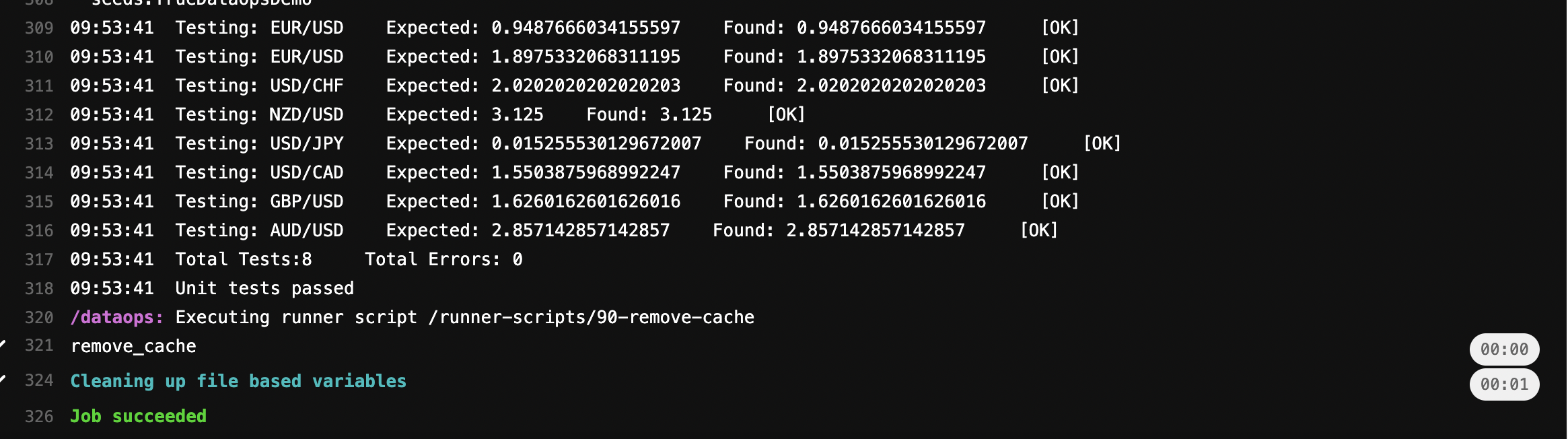And if failing: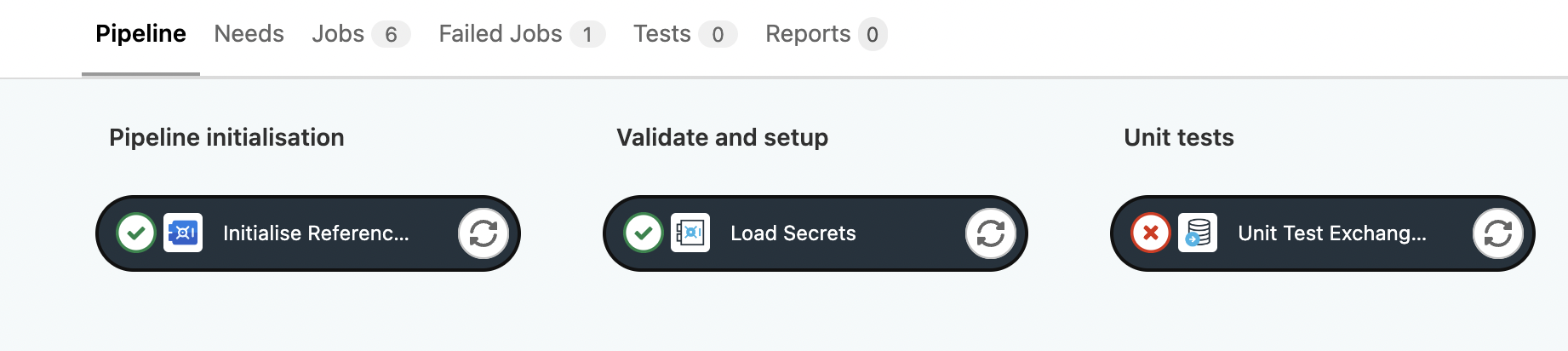with job details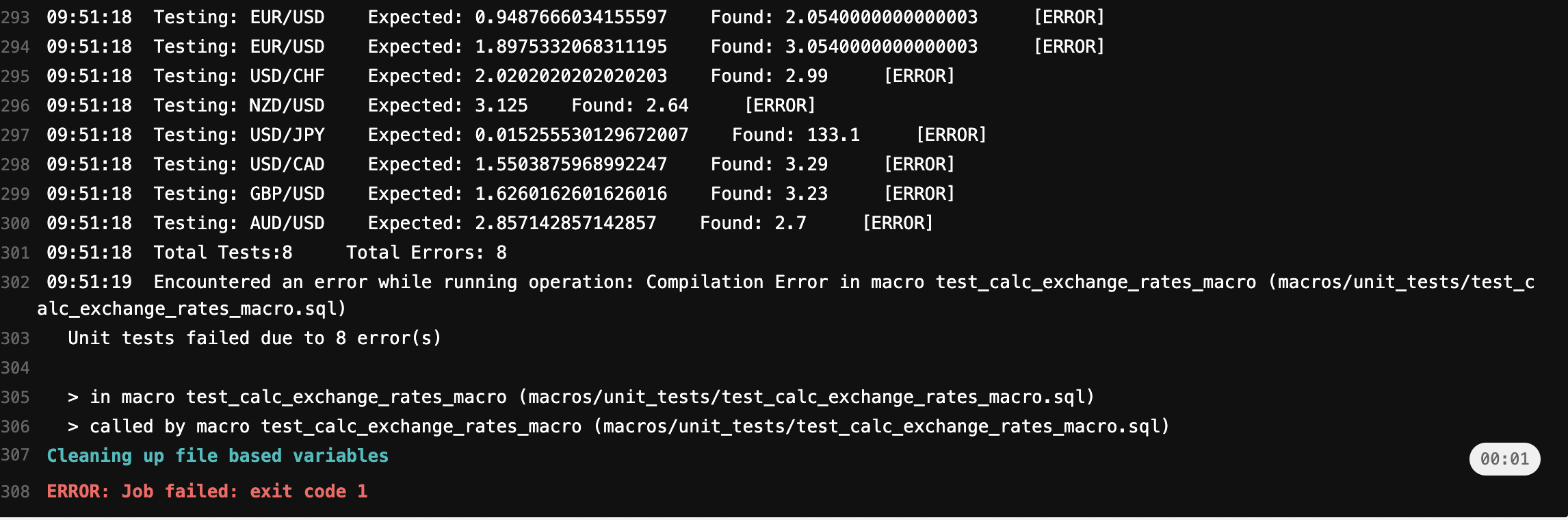## Test models​

This approach is a little more involved but is a much better approach when we have functions that operate on large amounts of data.

Conceptually we create a set of test data with known inputs and outputs, build a model on this using the Function we wish to test and then a second model with the expected outputs and then compare the two: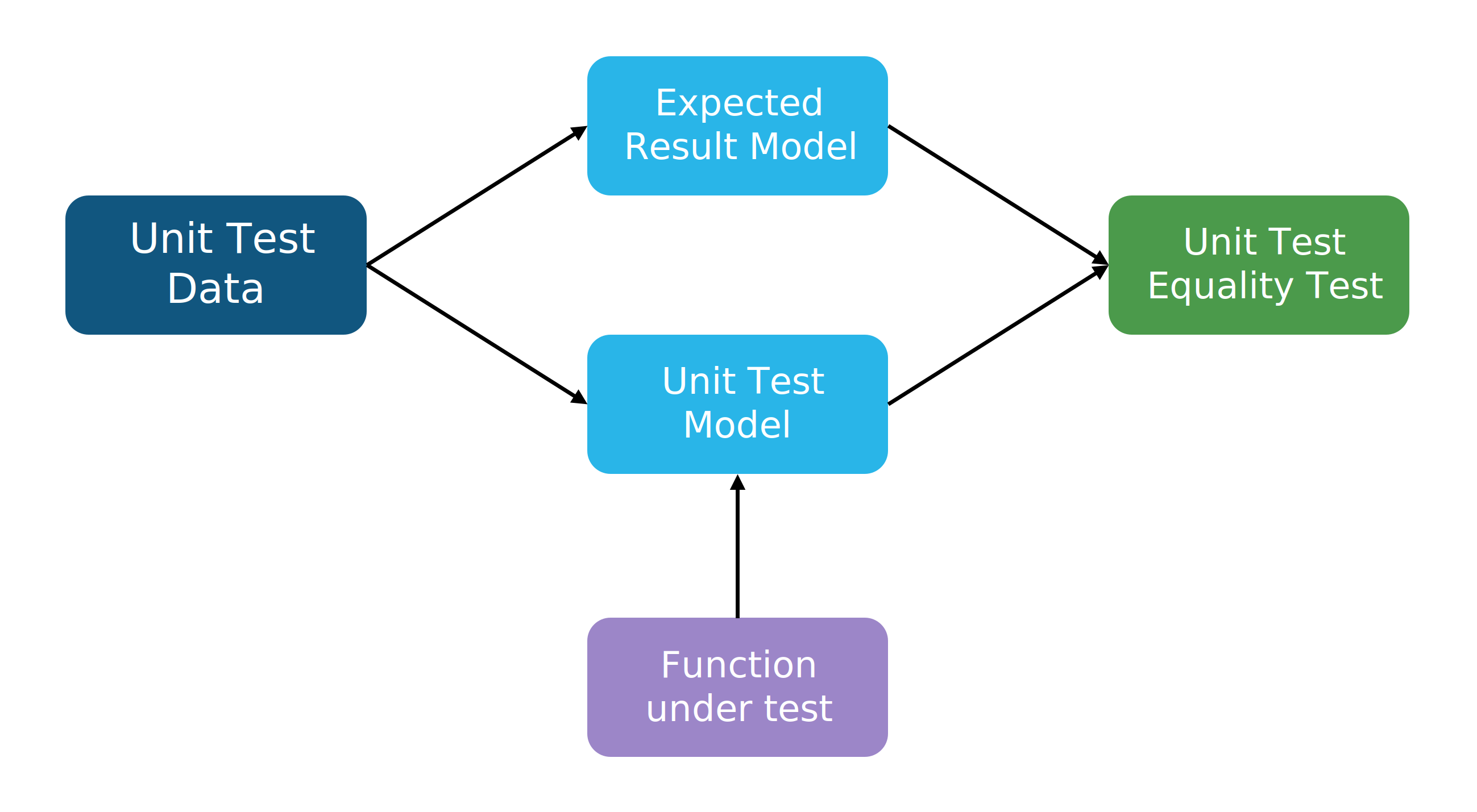If they exactly match, the tests pass, if there are any differences, the tests fail.

Consider we have a Snowflake function that multiplies two numbers together:

``create or replace function SHARED_FUNCTIONS.multiply_two_numbers(a float, b float)  returns float  comment='multiply two numbers'  as 'a * b';``

Which we would manage through SOLE like:

dataops/snowflake/database.template.yml
``databases:  <database-name>:    schemas:      SHARED_FUNCTIONS:        grants:          ALL PRIVILEGES:            - DATAOPS_WRITER            - DATAOPS_READER            - DATAOPS_ADMIN            - PUBLIC        functions:          multiply_two_numbers:            comment: "multiply two numbers"            language: SQL            return_type: NUMBER            return_behavior: IMMUTABLE            null_input_behavior: "RETURNS NULL ON NULL INPUT"            statement: "a * b"            arguments:              A:                type: NUMBER              B:                type: NUMBER            grants:              ALL PRIVILEGES:                - DATAOPS_WRITER``

We now want to test this against some known good cases.

First, we will create a CSV file with our test cases:

/dataops/modelling/seeds/unit_tests/multiply_two_numbers_data.csv
``input_1,input_2,expected_output2.0,2.0,4.04,2,81.5,2,3.02.5,50,1250.5,200,100``

and a seed configuration file to detail how we load this:

/dataops/modelling/seeds/unit_tests/multiply_two_numbers_data.yml
``version: 2seeds:  - name: multiply_two_numbers_data    config:      column_types:        input_1: float        input_2: float        expected_output: float``
Data Types

Note in some situations this file isn't necessary, Snowflake will infer column data types. However, when running unit tests, it's very common for a test that looks right to fail because somewhere along the line a data type wasn't cast the way you expected. This is a great example of the value of unit tests - they will find situations where you need explicit data type casting to make everything work the way you want.

We also need to include some configuration in our dbt_project file:

/dataops/modelling/dbt_project.yml
``models:  +transient: true  +materialized: table  TrueDataOpsDemo:    samples:      +schema: SAMPLES    unit_tests:      +schema: unit_tests      tags:        - "unit_tests"      +quote_columns: falseseeds:  +transient: true  TrueDataOpsDemo:    unit_tests:      +schema: unit_tests      tags:        - "unit_tests"      +quote_columns: false``

This simply defines the schema we should load our data into and tags it unit_tests so we can run all our unit tests together easily.

Now we need a DataOps job to load this data:

``Load Unit Test Data:  extends:    - .modelling_and_transformation_base    - .agent_tag  stage: Unit Test Prep  variables:    TRANSFORM_ACTION: SEED  script:    - /dataops || true  icon: \${INSERT_ICON}``

### Creating unit test models​

We need two models here, one that exercises our function and one to extract the expected output.

This model simply applies our Snowflake function to the two inputs for each row in the test file:

/dataops/modelling/models/unit_tests/multiply_two_numbers/test_function.sql
``select  SHARED_FUNCTIONS.multiply_two_numbers(input_1,input_2)::FLOAT as resultfrom {{ ref('multiply_two_numbers_data') }}``
Data Types

Again, note the Data Type casting

For reference, the results of this model when executed is:And a very simple one to extract the expected output into the same format. A

/dataops/modelling/models/unit_tests/multiply_two_numbers/expected_result.sql
``select  expected_output::FLOAT as resultfrom {{ ref('multiply_two_numbers_data') }}``
Data Types

Again, note the Data Type casting

For reference, the results of this model when executed is:We now need a DataOps job to build these 2 models:

``Build Unit Test Models:  extends:    - .modelling_and_transformation_base    - .agent_tag  variables:    TRANSFORM_ACTION: RUN    TRANSFORM_MODEL_SELECTOR: "tag:unit_tests"  stage: Unit Test Build  script:    - /dataops  icon: \${TRANSFORM_ICON}``

### Creating the final unit tests​

Now we have rendered models, the one generated from our well-defined inputs and put through the function under test, and one created from the expected outputs.

The actual unit test is now extremely simple - we just need to test whether these two models have identical data, which we do with a model test:

/dataops/modelling/models/unit_tests/multiply_two_numbers/expected_result.yml
``version: 2models:  - name: expected_result    tests:      - dbt_utils.equality:          compare_model: ref('unit_test')``

And that's it - we are just testing equality across our calculated and expected models. In this case, the test is attached to the expected_result and references the unit_test but it would work equally well the other way around.

Finally, a DataOps job to run this:

``Execute Unit Test Models:  extends:    - .modelling_and_transformation_base    - .agent_tag  variables:    TRANSFORM_ACTION: TEST    TRANSFORM_MODEL_SELECTOR: "tag:unit_tests"  stage: Unit Test Execute  script:    - /dataops  icon: \${TESTING_ICON}  artifacts:    when: always    reports:      junit: \$CI_PROJECT_DIR/report.xml``

### Pulling all this together​

When running all of the above, we get a pipeline segment that looks like this: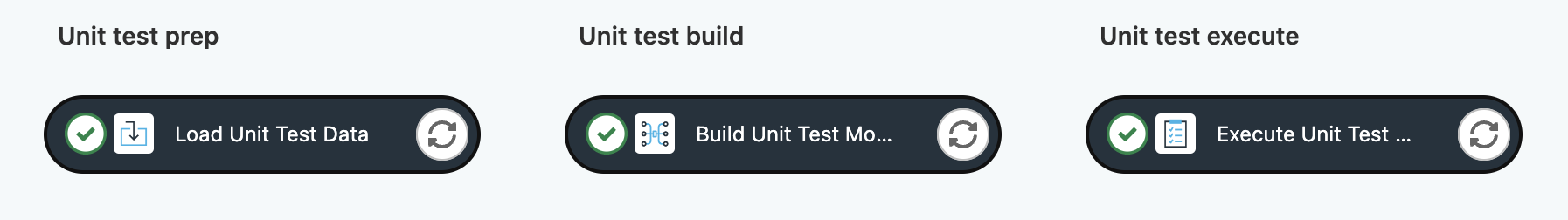And our unit test results are included in the DataOps Tests tab:### What does failure look like​

Let's consider in the future that someone decides to change our function to something incorrect e.g.

``create or replace function SHARED_FUNCTIONS.multiply_two_numbers(a float, b float)  returns float  comment='multiply two numbers'  as 'a + b';``

When a pipeline in `dev` or any `feature branch` runs, the unit test data is loaded, and the models are built, but the tests fail and the pipeline is stopped:With appropriate reporting:### Extended to more unit tests​

Since all of the DataOps jobs refer to the unit_tests tag, there is no need to ever touch or extend these jobs again, we can simply add more:

• Unit test data files
• Unit test models
• Unit test equality tests

Once done a few times it should take no more than 20 minutes to add unit tests to a simple/moderate complexity function.

### Testing other types of function​

In this example, we have tested a simple SQL function, but the same principles can be used to test:

• Any SQL UDF
• Any Java UDF
• Any Python UDF
• Any functions created by Snowpark (Java, Scala or Python)